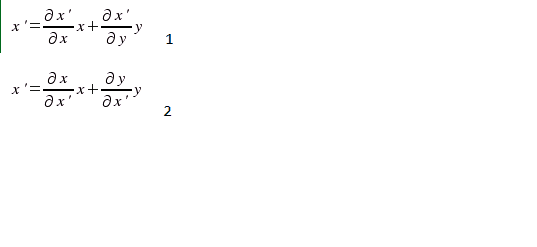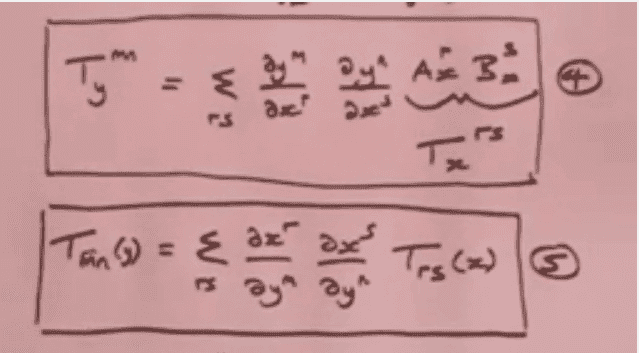# Covariant equation intuition confusing me

NotASmurf
Hey all, I've just started tensor analysis but do not understand why in contravarient uses 1 and covarient uses 2, could someone please explain these? Perhaps my understanding of the definitions is causing me to misunderstand why its written like this. Any help appreciated.Gold Member
Can you explain your notation? What are "x',y',x,y" supposed to be in this picture?

NotASmurf
"The components of a vector in two dimensions are defined in the literature in relation to a change of coordinates from (x,y) to (x',y'), say. The contravariant components are those which transform as follows e.g. for the new coordinate x' in terms of the old (x,y):"

Gold Member
"The components of a vector in two dimensions are defined in the literature in relation to a change of coordinates from (x,y) to (x',y'), say. The contravariant components are those which transform as follows e.g. for the new coordinate x' in terms of the old (x,y):"

That is some seriously confusing notation. What book is this?

A change of coordinates from (x,y) to (x',y') looks simply like ##x'=x'(x,y),~y'=y'(x,y)## so these are simply functions of the old coordinates. Vector and one form components transform similarly to the picture you uploaded, but I've never seen that kind of notation. Usually the notation is such that for a vector: $$V^{\alpha'} = \sum_\beta \frac{\partial x^{\alpha'}}{\partial x^\beta} V^\beta$$ And for a one form: $$\omega_{\alpha'}=\sum_\beta \frac{\partial x^\beta}{\partial x^{\alpha'}}\omega_\beta$$

Staff Emeritus
Gold Member
Those equations and the corresponding ones for y' define covariant and contravariant in this (obsolete and horrible) approach to tensors, so it's a bit odd to ask why the equations look the way they do. It does however make sense to ask why those terms are used. The idea is that in the covariant case, the matrix that transforms ##\begin{pmatrix}x\\ y\end{pmatrix}## to ##\begin{pmatrix}x'\\ y'\end{pmatrix}## is the same matrix that transforms the basis vectors (hence the term covariant), and in the contravariant case, the matrix that does the transformation is the inverse of the matrix that transforms the basis vectors (hence the term contravariant).

The modern approach starts with a vector space V. Its dual space V* is defined as the vector space of linear maps from V into ℝ. Now suppose that ##(e_1,\dots,e_n)## and ##(e_1',\dots,e_n')## are ordered bases for V. There must exist numbers ##M^j_i## such that ##e_i'=M^j_i e_j## (each of the primed basis vectors can be expressed as a linear combination of the unprimed basis vectors). Now we can examine the relationship between the components of an arbitrary ##v\in V## with respect to these two ordered bases. It turns out that it's given by ##v^i{}'=(M^{-1})^i_j v^j##. Because of this, the n-tuple of components is said to "transform contravariantly".

Edit: I fixed a typo in the paragraph above after it was pointed out by Matterwave below.

There's a simple way to use an ordered basis for V to define an ordered basis for V*. Because of this, it makes sense to ask for the relationship between the components of an arbitrary ##f\in V^*## with respect to the two ordered basis for ##V^*## that are defined from the two ordered bases for ##V## mentioned above. It turns out that the relationship is given by ##f_i'=M_i^j f_j##. Because of this, the n-tuple of components is said to "transform covariantly".

You can find many of the details of this approach in this post. I also recommend chapter 3 in "A first course in general relativity" by Schutz. It's a nice introduction to this stuff.

Last edited:
•smodak and NotASmurf
NotASmurf
Thank you so much.

Gold Member
There must exist numbers ##M^j_i## such that ##e_i'=M^j_i e_i##

I believe you mean ##e_i'=M^j_i e_j## :)

•NotASmurf
NotASmurf
in the contra-variant case, the matrix that does the transformation is the inverse of the matrix that transforms the basis vectors
Confusion, in the video teaching me this they say that
equation 4 is the contravarient one and that equation 5 (the inverse) is the covariant one, your intuition made sense but this confused me now, his notation isn't helping.Also covarient is the one that is reference frame independent right? Apologies for newbie-ness.

Gold Member
See post #4, "contra variant vector" is the old term for "vector" and "co variant vector" is the old term for "one form". Contra variant tensors have indices up and co variant tensors have indices down.

All tensors are "reference frame independent", in the appropriate sense.

•NotASmurf
Staff Emeritus
Gold Member
Confusion, in the video teaching me this they say that
equation 4 is the contravarient one and that equation 5 (the inverse) is the covariant one, your intuition made sense but this confused me now, his notation isn't helping.
In my notation, the first one is ##T_y^{mn}=(M^{-1})^m{}_r(M^{-1})^n{}_s T_x^{rs}## and the second one is ##T_{mn}(y)=M^r{}_m M^s{}_n T_{rs}(x)##.

Also covarient is the one that is reference frame independent right?
Elements of ##V## and elements of ##V^*## are all independent of the frame (=the ordered basis). But their components with respect to an ordered basis are not.

Staff Emeritus
Gold Member
I believe you mean ##e_i'=M^j_i e_j## :)
Yes, thanks. I wrote that post very quickly. I have edited that typo now. Also, it would have been even better to write ##e_i'=M^j{}_i e_j##, because when we start using the metric to raise and lower indices, we're going to have to keep track of the horizontal positions of the indices.

NotASmurf
See post #4, "contra variant vector" is the old term for "vector" and "co variant vector" is the old term for "one form". Contra variant tensors have indices up and co variant tensors have indices down.

All tensors are "reference frame independent", in the appropriate sense.
one form? what does that mean?
Thanks for help so far, I liked your definition of contravarient.

Staff Emeritus
Gold Member
one form? what does that mean?
Thanks for help so far, I liked your definition of contravarient.
There's a vector space ##T_pM## associated with each point ##p## in a smooth manifold ##M##. An element of ##T_pM## is called a tangent vector at ##p##. An element of ##T_pM^*## is called a cotangent vector at ##p##. A vector field on ##M## is a function that associates a tangent vector at ##p## with each ##p\in M##. A cotangent vector field on ##M## is a function that associates a cotangent vector at ##p## with each ##p\in M##. A cotangent vector field is also called a 1-form (for reasons that I will not go into here).